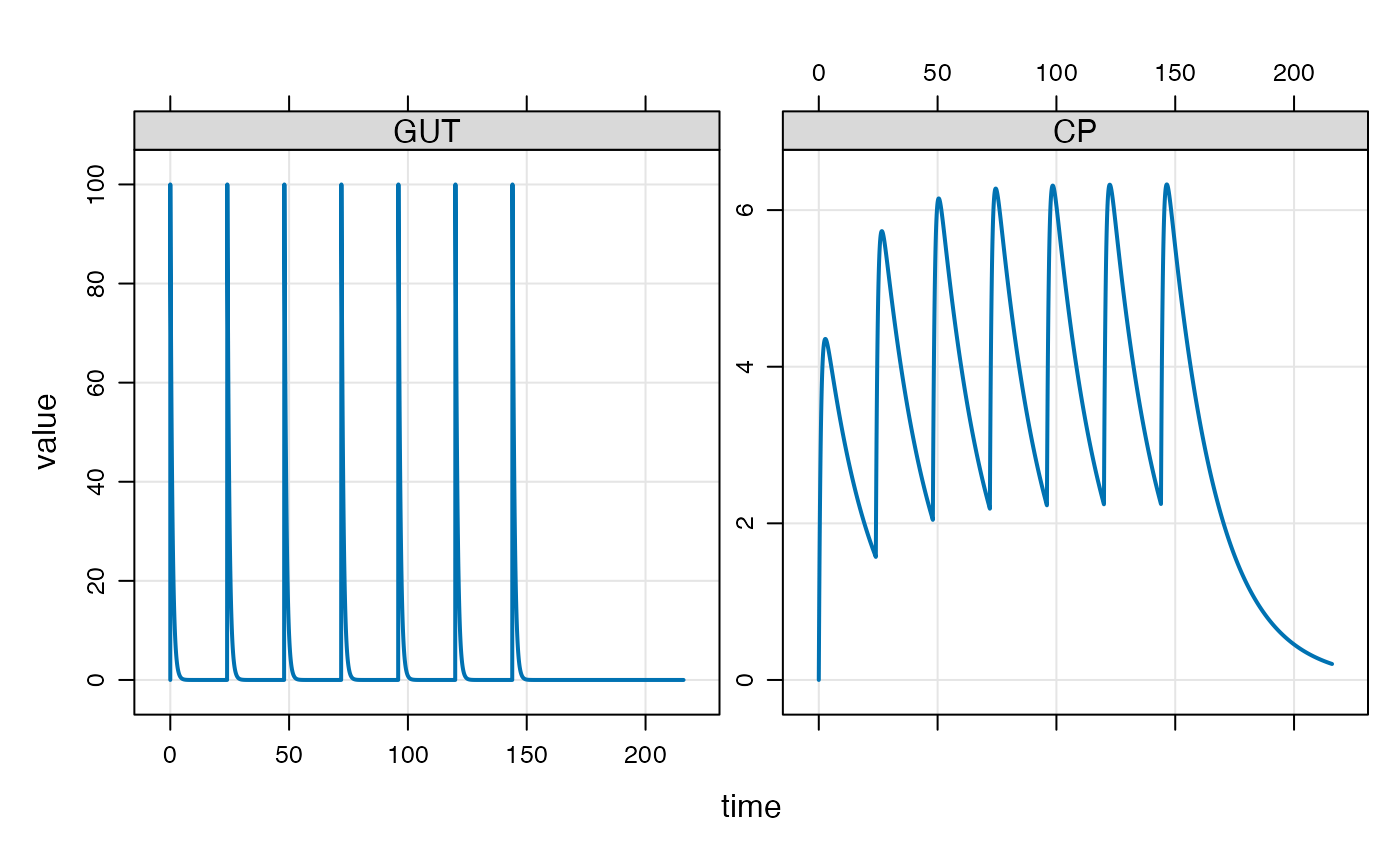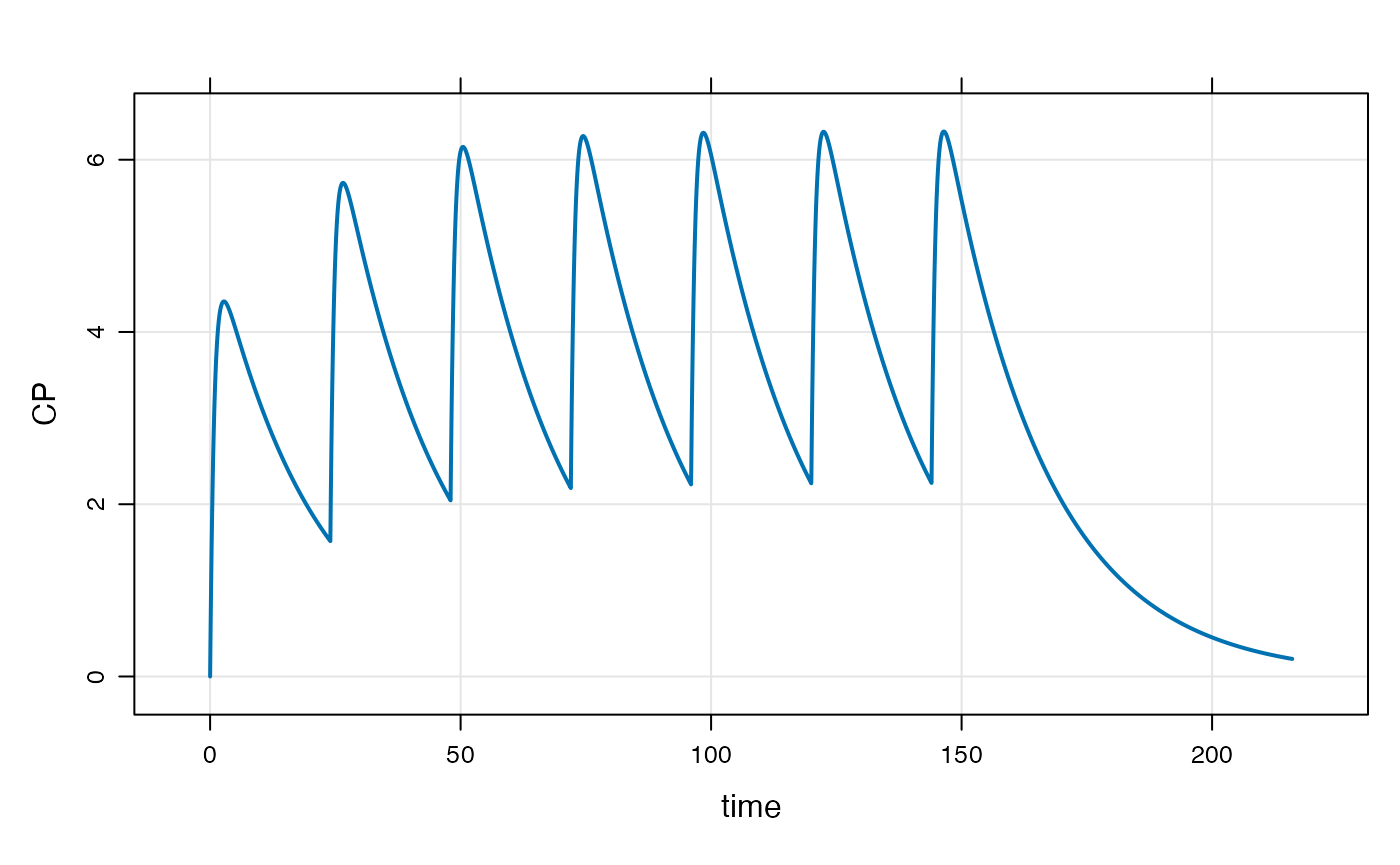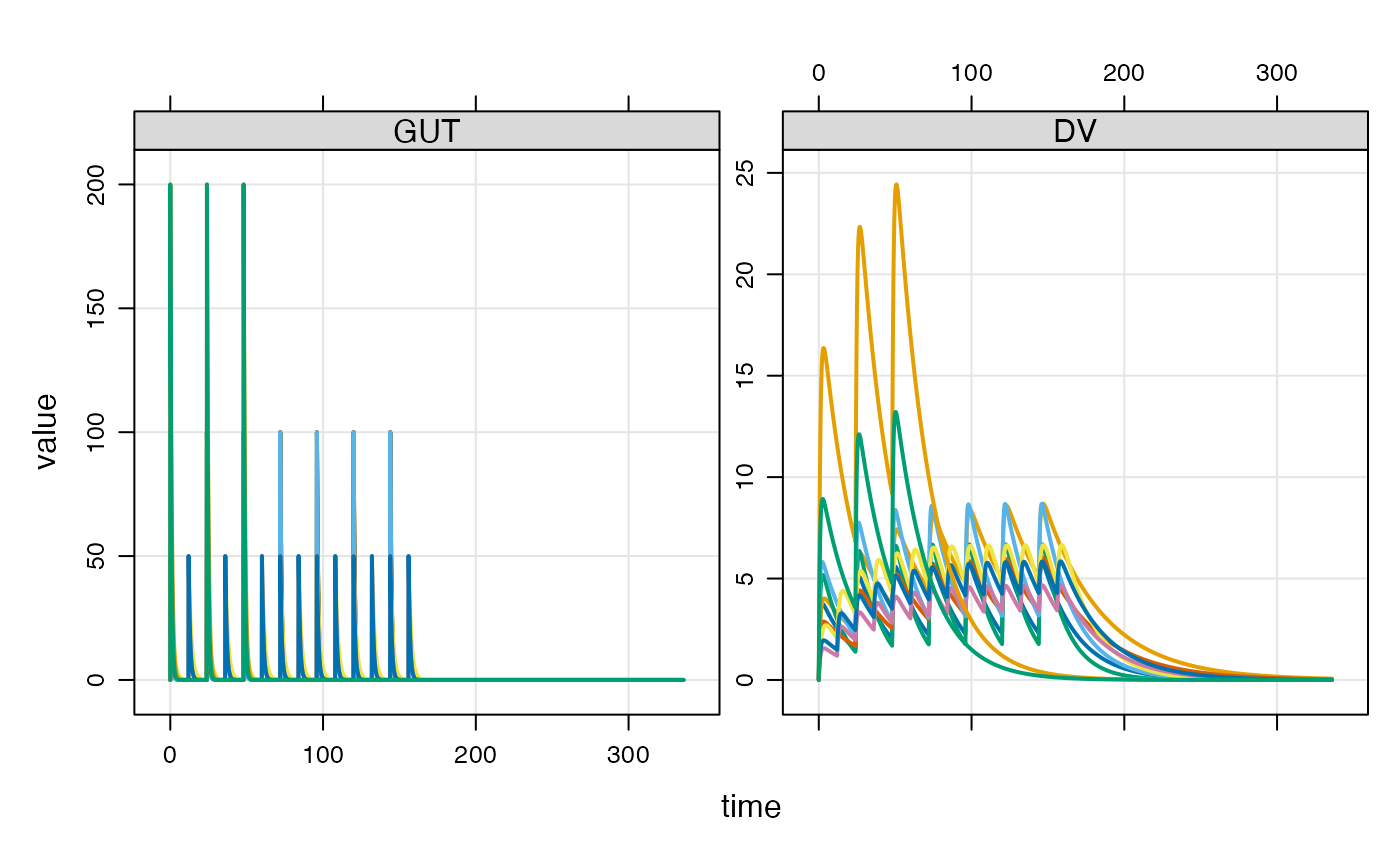library(mrgsolve)
library(dplyr)

## Introduction

Event objects are simple ways to implement PK dosing events into your model simulation.

Let’s illustrate event objects with a one-compartment, PK model. We read this model from the mrgsolve internal model library.

mod <- house(end = 216, delta = 0.1)

## Events

Events are constructed with the ev() function

e <- ev(amt = 100, ii = 24, addl = 6)

This will implement 100 unit doses every 24 hours for a total of 7 doses. e has class ev, but really it is just a data frame

e
. Events:
.   time amt ii addl cmt evid
. 1    0 100 24    6   1    1
as.data.frame(e)
.   time amt ii addl cmt evid
. 1    0 100 24    6   1    1

We can implement this series of doses by passing e in as the events argument to mrgsim

mod %>% mrgsim(events = e) %>% plot(GUT + CP ~ time)The events can also be implemented with the ev constructor along the simulation pipeline

mod %>%
ev(amt = 100, ii = 24, addl = 6) %>%
mrgsim %>%
plot(CP ~ time)### Event defaults and expectations

1. amt is required
2. evid = 0 is forbidden
3. Default time is 0
4. Default evid is 1
5. Default cmt is 1

Also by default, rate, ss and ii are 0.

## Combine events

mrgsolve has operators defined that allow you to combine events. Let’s first define some event objects.

e1 <- ev(amt = 500)
e2 <- ev(amt = 250, ii = 24, addl = 4)
e3 <- ev(amt = 500, ii = 24, addl = 0)
e4 <- ev(amt = 250, ii = 24, addl = 4, time = 24)

We can combine e1 and e3 with a collection operator

c(e1, e4)
. Events:
.   time amt cmt evid ii addl
. 1    0 500   1    1  0    0
. 2   24 250   1    1 24    4

mrgsolve also defines a seq() method that lets you execute one event and then a second event

seq(e3, e2)
. Events:
.   time amt ii addl cmt evid
. 1    0 500 24    0   1    1
. 2   24 250 24    4   1    1

Notice that e3 has both ii and addl defined. This is required for mrgsolve to know when to start e2.

### Combine to create a data set

We can take several event objects and combine them into a single simulation data frame with the as_data_set function.

e1 <- ev(amt = 100, ii = 24, addl = 6,  ID = 1:5)
e2 <- ev(amt = 50,  ii = 12, addl = 13, ID = 1:3)
e3 <- ev(amt = 200, ii = 24, addl = 2,  ID = 1:2)

When combined into a data set, we get * N=5 IDs receiving 100 mg Q24h x7 * N=3 IDs receiving 50 mg Q12h x 14 * N=2 IDs receiving 200 mg Q48h x 3

data <- as_data_set(e1, e2, e3)

data
.          ID time cmt evid amt ii addl
. 1...1     1    0   1    1 100 24    6
. 1.1...2   2    0   1    1 100 24    6
. 1.2...3   3    0   1    1 100 24    6
. 1.3       4    0   1    1 100 24    6
. 1.4       5    0   1    1 100 24    6
. 1...6     6    0   1    1  50 12   13
. 1.1...7   7    0   1    1  50 12   13
. 1.2...8   8    0   1    1  50 12   13
. 1...9     9    0   1    1 200 24    2
. 1.1...10 10    0   1    1 200 24    2

To simulate from this data set, we use the data_set function. First, let’s add an OMEGA matrix to this model:

mod <- omat(mod, as_dmat(c(0.1, 0.1, 0.1, 0.1)))
mod %>% data_set(data) %>% mrgsim(end=336) %>% plot("GUT, DV")